Your No1 source for Latest Entrance Exams, Admission info

 Score 100 percent in English Grammar! Special Course by English Academy Experts - 55 Lessons, 40 Tests for Everyone in CBSE Class 6 to 10 Students - Check out Special Offer englishacademy.successcds.net

Home >> Class 10 >> Science >>

# Electricity Important Questions 1, 2 and 5 Marks CBSE Class 10 Science Chapter 12

## NCERT Solutions for Class 10 Science Chapter 12 “Electricity” Important Questions

Here are the Electricity important questions of 1,2,3 and 5 Marks for CBSE Class 10 Science Chapter 12. The important questions we have compiled will help the students to brush up on their knowledge about the subject. Students can practice Class 10 Science important questions to understand the subject better and improve their performance in the board exam. The solutions provided here will also give students an idea about how to write the answers. Take Free Online MCQ Test for Class 10

### Electricity Important Question for CBSE Class 10 Science Chapter 12

Electricity Important Questions Video

 Electricity MCQs 1 Marks Important Questions 2 Marks Important Questions 5 Marks Important Questions

Electricity Class 10 Science MCQs (1 Mark)

1. A battery of 10 volt carries 20,000 C of charge through a resistance of 20 Ω. The work done in 10 seconds is

(a) 2 × 103 joule
(b) 2 × 105 joule
(c) 2 × 104 joule
(d) 2 × 102 joule

Answer - b

Explanation: (b) W= qV= 20000 × 10 = 2,00, 000 = 2 × 105 J

2. To get 2 Ω resistance using only 6 Ω resistors, the number of them required is
(a) 2
(b) 3
(c) 4
(d) 6

Answer - b

Explanation: (b) Three resistors of 2 Ω is required to get 6 Ω because resultant is more than individual so they all must be connected in series.

3. The temperature of a conductor is increased. The graph best showing the variation of its resistance is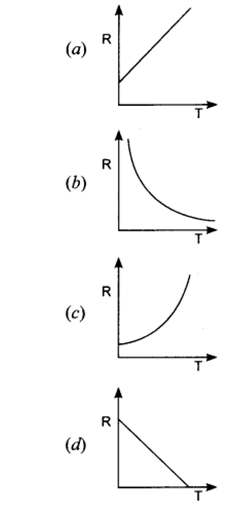Answer - A

Explanation: (a) Resistance is directly proportional to temperature of the conductor.

4. A boy records that 4000 joule of work is required to transfer 10 coulomb of charge between two points of a resistor of 50 Ω. The current passing through it is
(a) 2 A
(b) 4 A
(c) 8 A
(d) 16 A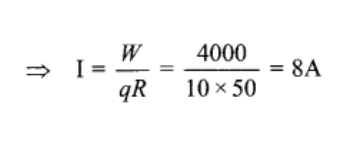Answer - c

Explanation : (c) Work done in transferring the charge W= qV = qlR …….. (V = IR)

5. The resistance whose V-I graph is given below is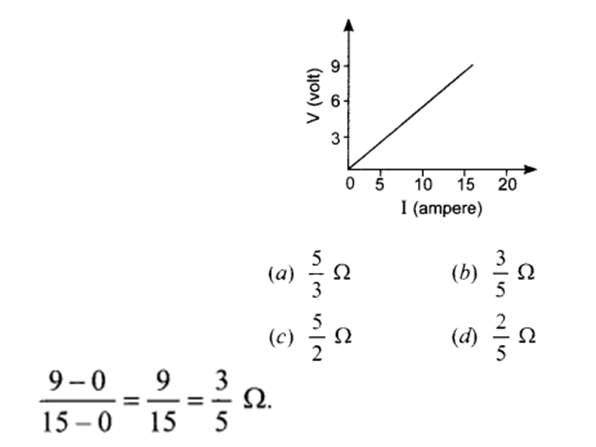Answer - b

Explanation: (b) Resistance = slope line of V-I graph =

### Electricity Class 10 Science 1 Mark Important Questions

Q1. - Define electric potential.
Answer - Electric potential at a point in an electric field is defined as the work done in moving a unit positive charge from infinity to that point in the electric field.

Q2.  - State the relation between work (W), change (q) and electric potential (V).
Answer - V = W/q.

Q3.  - Define 1 volt electric potential.
Answer - Electric potential is said to be 1 volt if 1 Joule of work is done in moving 1 coulomb charge from infinity to a point in the electric field.

Q4. - Is electric potential a scalar or a vector physical quantity ?
Answer - Electric potential is a scalar physical quantity.

Q5. - What is meant by potential difference between two points ?
Answer - Work done per unit charge in moving a unit positive charge from one point to another point in an electric field is called potential difference between two points.

Q6. - State the relation between the current I passing in a conductor, number of electrons (n) flowing through any cross-section of the conductor, magnitude of charge on an electron (e) and time t.
Answer - I = ne/t.

Q7.  - Define 1 ampere electric current.
Answer - Electric current through a conductor is said to be 1 ampere if 1 coulomb electric charge flows through a cross-section of a conductor in 1 second.

Q8. - How is the direction of electric current related to the direction of flow of electrons in a wire ?
Answer - The direction of electric current in a wire is just opposite to the direction of flow of electrons in the wire.

Top

### Electricity Class 10 Science 2 Marks Important Questions

Q1. - State the law that gives the relationship between the potential difference (V) across the two ends of a conductor and the current (I) flowing through it.
Answer - Ohm’s law states that the electric current flowing through a conductor is directly proportional to the potential difference across the ends of the conductor, provided the temperature and . other physical conditions of the conductor remain the same.

Q2. - The voltage - current (V-I) graph of a metallic circuit at two different temperature T1 and T2 is shown. Which of the two temperatures is higher and why ?
Or
The voltage-current (V-I) graph of a metallic conductor at two different temperatures T1 and T2 is shown in figure. At which temperature is the resistance higher?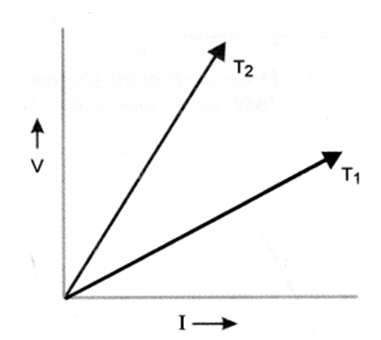Answer -  Slope of I-V graph = resistance of metallic conductor.
Since, slope of I-V graph at temperature T2 is greater than the slope of I-V graph at temperature T1,  therefore, resistance at T2 is greater than resistance at T1 . Since, resistance of a metallic conductor Increases with increase in temperature, therefore, T2 > T1.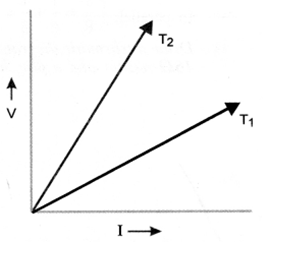Q3. - How does the resistance of a wire vary with its cross-section area?

Answer - Resistance of a wire is inversely proportional to its cross-sectional area. More is cross-sectional area of a conductor, less is the resistance of the conductor.Q4. - State Joule’s law of heating.

Answer - According to Joule’s law of heating, the amount of heat produced in a conductor is directly proportional to the square of electric current passing through the conductor, directly proportional to the resistance of the conductor, and directly proportional to the time for which electric current passes through the conductor.

Q5. - List two differences between a voltmeter and ammeter.
Answer -

 Ammeter Voltmeter 1. Ammeter measures electric current in the circuit. 2. Ammeter is connected in series in an electric circuit. 1. Voltmeter measures the potential difference be­tween two points on a conductor. 2. Voltmeter is connected in parallel across the ends of a conductor or resistor.

Q6. Two wires, one of copper and other of manganin, have equal lengths and equal resistances. Which wire is thicker ? Given that resistivity of copper is lower than that of manganin.
Ans - Since l and R of both the wires are same, so A p . Since, resistivity ip) of manganin is higher than that of copper, so manganin wire is thicker than that of copper wire.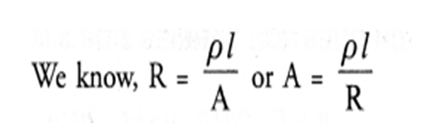Q7. - In the circuit diagram shown, the two resistance wires A and B are of the same length and same material,but A is thicker than B. Which ammeter Af or A2 will indicate higher reading for current ? Give reason.

Answer - The current will be high if resistance is low. p and l for both wires A & B are same but area of cross-section (A) of wire A is- more than the wire B. Therefore, the resistance of wire A is less than the resistance of wire B. Hence, ammeter A connected in series with the wire A will indicate higher reading for current.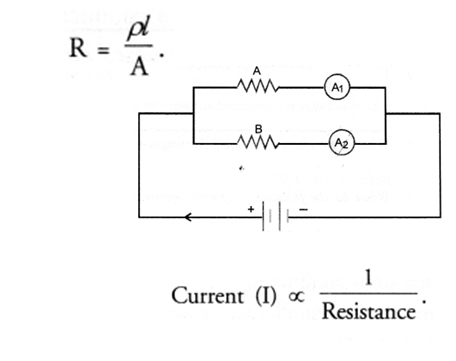Q8. - Two students perform experiments on two given resistors R1 and R2 and plot the following V-I graphs. If R1 > R2, which of the two diagrams correctly represent the situation on the plotted curves ? Justify your answer.

Answer - Resistance of a conductor = slope of I-V graph. It means, resistance is high if slope of I-V graph for it is steeper.
Since R1 > R2, therefore, slope of I-V graph for R1 must be steeper than the slope of I-V graph for R2 Thus, diagram I represents the situation correctly.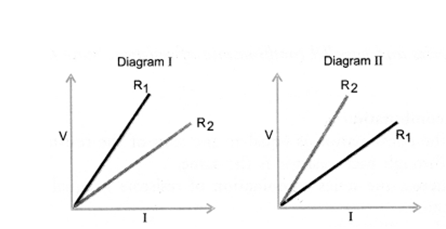Q9. - Draw a schematic diagram of a circuit consisting of a battery of 12V, three resistors of 5Ω, 10Ω and 20Ω connected in parallel, an ammeter to measure the total current through the circuit, voltmeter to measue the potential difference across the combination of resistors. [CBSE (All India) 2008]

Answer -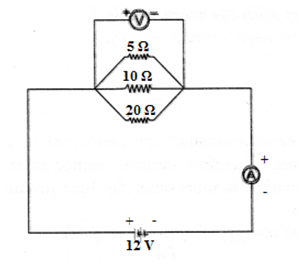Q10. (a) Why an ammeter likely to bum out if you connect it in parallel ?

(b) Why is series arrangement not found satisfactory for domestic lights

Answer - (a) Ammeter is a low resistance device. If it is connected in parallel, a large current flows through it. Hence, large heat is produced and it may burn the ammeter.
(b) If domestic lights are connected in series, then all lights will be switched off even when only one light fuses.

Important Videos Links

Top

### Electricity Class 10 Science 5 Mark Important Questions

Q1 - Describe an experiment to study the factors on which the resistance of a conductor or a conducting wire depends.

Ans - For Experiment: Connect the various electrical components as shown in figure 15.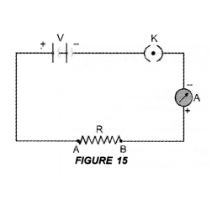Dependence of Length of a Conductor:

Take a copper wire of length l and connect it between the terminals A and B. Note the reading of ammeter. Now take another copper wire of same area of cross-section but of length 2l. Connect it between the terminals A and B by disconnecting the previous wire. Again, note the reading of ammeter. It will be found that the reading of ammeter (i.e., electric current) in the second case is half of the reading of ammeter in the first case.Thus, resistance of a conductor is directly proportional to the length of the conductor, i.e. resistance ∝ length of the conductor.
Thus, more is the length of a conductor, more is its resistance. Thus, the resistance of’a conductor is ‘inversely proportional to the area of cross -section of the conductor.Dependence on area of Cross-Section of a Conductor:

Now take two copper wires of same length but of different area of cross-sections. Let area of cross-section of first wire is more than the area of cross-section of the second wire. Connect first wire between the terminals A and B in the circuit shown in figure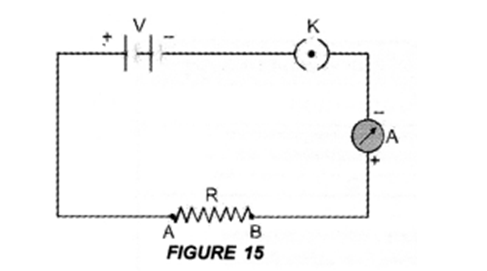1. Note the reading of ammeter :

Now disconnect the first wire and connect the second wire between the terminals A and B. Again note the reading of the ammeter. It will be found that the reading of ammeter (i.e. electric current) is more when first wire (i.e. thick wire) is connected between A and B than the reading of the ammeter when second wire (i.e. thin wire) is connected between the terminals A and B.
Thus, the resistance of a thin wire is more than the resistance of a thick wire.

2. Effect of the Nature of Material:

Take two identical wires, one of copper and other of aluminium. Connect the copper wire between the terminals A and B.
3. Note the reading of ammeter. Now, connect the aluminium wire between the terminals A and B. Again note the reading of ammeter.
4. It is found that the reading of ammeter when copper wire is connected in the circuit is more than the reading of the ammeter when aluminium wire is connected in the circuit.
Therefore, resistance of copper wire is less than the resistance of aluminium wire. Hence, resistance of a wire or a conductor depends upon the nature of the material of the conductor.

3. Effect of Temperature of Conductor:

If the temperature of a metallic conductor connected in the circuit increases, its resistance increases.
Thus, factors on which resistance of a conductor depends are:
1. its length,
2. its area of cross-section,
3. the nature of its material and
4. its temperature.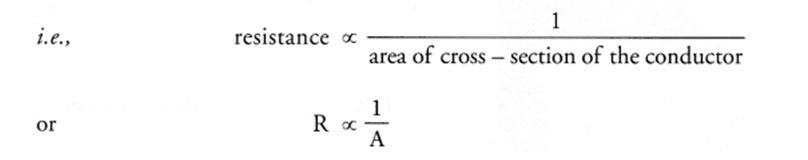Q2. - What is Joule’s heating effect? List applications of Joule’s heating effect in daily life.

Answer - Joule’s law can be stated as : The amount of heat produced in a conductor is (i) directly proportional to the square of the electric current flowing through it.
This is  H ∝ I2  -
(ii) directly proportional to the resistance of the conductor or resistor.
That is,  H ∝ R ‘
(iii) directly proportional to the time for which the electric current flows through the conductor or resistor.
That is,  H ∝ t
Combining (i), (ii) and (iii), we get H ∝ I2 Rt.
Or  H = KI2Rt, where K is constant of proportionality
If  K = 1, then H = I2Rt joule
This is known as Joule’s law of heating.

Four Applications:
When electric appliances like electric heater, electric iron and water heater etc. are connected to the main supply of electricity, these appliances become hot but the connecting wires remain cold.
We know, the heat produced is directly proportional to the resistance of the material through which current flows. The element of the electric heater is made of nichrome. Since the resistance of nichrome is high, so a large amount of heat is produced in the element of the electric heater. However, heat produced in connecting wires made of copper or aluminium is very small and hence they are not heated up.

The filament of an electric bulb is made of a thin wire of tungsten. The melting point of filament is high i.e., about 3380 °C. The filament of the bulb is enclosed in a glass envelope fixed over insulated support as shown in figure 28. The glass envelope of the electric bulb is filled with inactive gases like nitrogen and argon to increase the life of the tungsten filament.
Since the resistance of the thin filament is very high, so a large heat is produced as the electric current flows through the filament. Due to this large amount of heat produced, the filament of the bulb becomes white-hot. Hence, the filament of the bulb emits light and heat.

An electric fuse is a safety device connected in series with the electric circuit. An electric fuse is a wire made of a material whose melting point is very low. Examples of the materials of making fuse wires are copper or tin-lead alloy. When a large current flows through a circuit and hence through a fuse wire, a large amount of heat is produced. Due to this large amount of heat, the fuse wire melts and the circuit is broken so that current stops flowing in the circuit. This saves the electric circuit from burning due to the passage of a large current through it.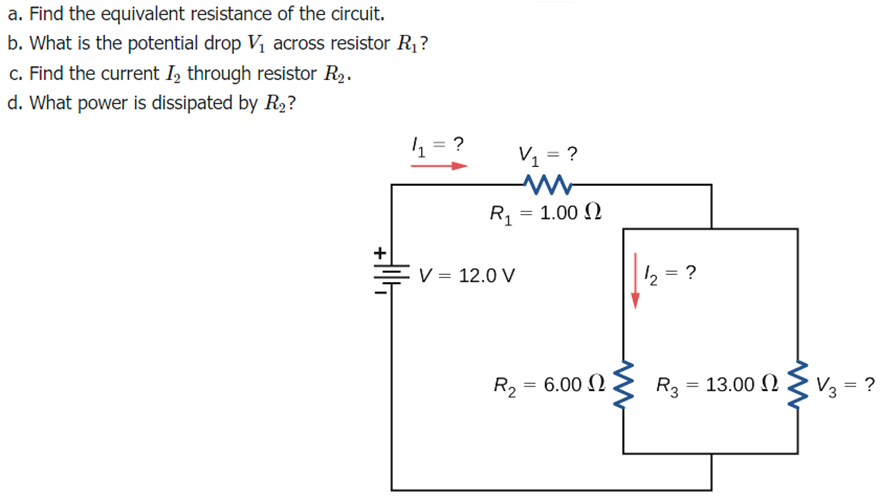Solution -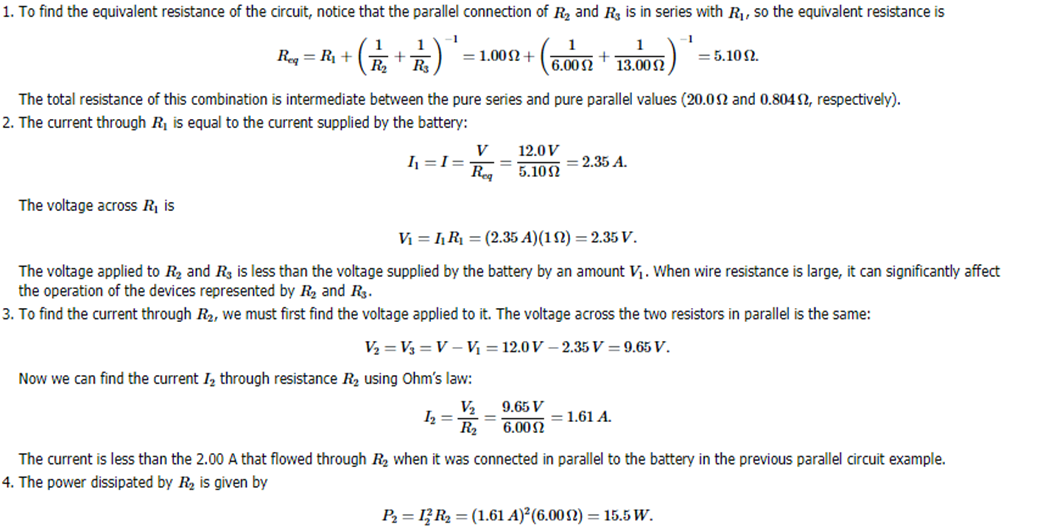Top

Important Questions Videos Links